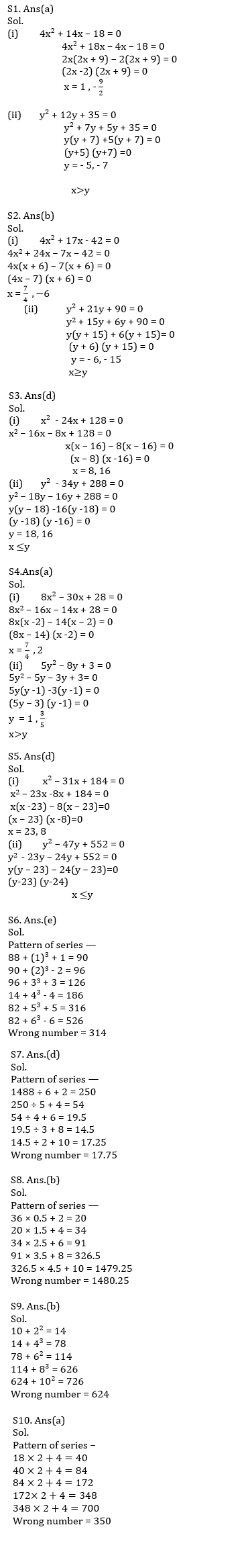Latest Banking jobs   »   Quantitative Aptitude Quiz For RRB PO...

# Quantitative Aptitude Quiz For RRB PO Mains 2021- 29th August

Directions (1-5): In each of these questions, two equations are given. You have to solve both the equations and give answer
(a) if x > y
(b) if x ≥ y
(c) if x < y
(d) if x ≤ y
(e) if x = y or no relation can be established between x and y.

Q1.
(i) 4x² + 14x – 18 = 0
(ii) y² + 12y + 35 = 0

Q2.
(i) 4x² + 17x – 42 = 0
(ii) y² + 21y + 90 = 0

Q3.
(i) x² – 24x + 128 = 0
(ii) y² – 34y + 288 = 0

Q4.
(i) 8x² – 30x + 28 = 0
(ii) 5y² – 8y + 3 = 0

Q5.
(i) x² – 31x + 184 = 0
(ii) y² – 47y + 552 = 0

Directions (6 – 10): Find the wrong number based of given pattern in the following number series:

Q6. 88, 90, 96, 126, 186, 314, 526
(a) 88
(b) 90
(c) 126
(d) 186
(e) 314

Q7. 1488, 250, 54, 19.5, 14.5, 17.75
(a) 1488
(b) 250
(c) 54
(d) 17.75
(e) 14.5

Q8. 36, 20, 34, 91, 326.5, 1480.25
(a) 36
(b) 1480.25
(c) 91
(d) 20
(e) 326.5

Q9. 10, 14, 78, 114, 624, 726
(a) 726
(b) 624
(c) 10
(d) 14
(e) 114

Q10. 18, 40, 84, 172, 350, 700
(a) 350
(b) 700
(c) 84
(d) 18
(e) 40

Solutions#### Congratulations!Union Budget 2023-24: Free PDF